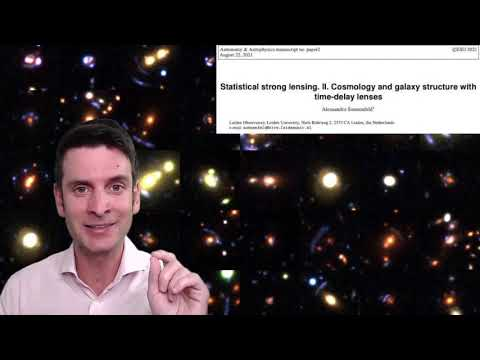# Deep quantum neural networks form Gaussian processes###### user

What are the proof techniques used by the authors?

AI: The text does not provide a clear answer to this question.###### user

What are the main results?

AI: The main results of the text are: 1. Certain models based on Haar random unitary or orthogonal deep QNNs converge to Gaussian processes in the limit of large Hilbert space dimension. 2. The derivation of this result is more nuanced than in the classical case due to the role played by the input states, the measurement observable, and the fact that the entries of unitary matrices are not independent. 3. The ensuing Gaussian processes cannot be used to efficiently predict the outputs of the QNN via Bayesian statistics. 4. Expectation values and gradients concentrate as $\mathcal{O}\left(\frac{1}{e^d \sqrt{d}}\right)$ -- exponentially in the Hilbert space dimension. 5. The concentration of measure phenomenon in Haar random QNNs is much worse than previously thought. 6. The results improve our understanding of concentration in $t$-designs.###### user

What is the variance of the Gaussian distribution?

AI: The text does not provide information about the variance of the Gaussian distribution.###### user

How is Carleman's condition used?

AI: The text does not mention anything about Carleman's condition, therefore, it cannot be used in this context.###### user

How does the variance of the Gaussian process when the circuit is Haar random over the unitary and orthogonal groups? Which case has the larger variance?

AI: The text does not provide information on the variance of the Gaussian process for Haar random quantum neural networks over the unitary and orthogonal groups, therefore it cannot be determined which case has the larger variance.###### user

How does the variance of the Gaussian process compare when the circuit is Haar random over the unitary and orthogonal groups? Which case has the larger variance?

AI: The text does not provide information on how the variance of the Gaussian process compares when the circuit is Haar random over the unitary and orthogonal groups, or which case has the larger variance.

## Authors

Diego García-Martín, Martin Larocca, M. Cerezo

## Abstract

It is well known that artificial neural networks initialized from independent and identically distributed priors converge to Gaussian processes in the limit of large number of neurons per hidden layer. In this work we prove an analogous result for Quantum Neural Networks (QNNs). Namely, we show that the outputs of certain models based on Haar random unitary or orthogonal deep QNNs converge to Gaussian processes in the limit of large Hilbert space dimension $d$. The derivation of this result is more nuanced than in the classical case due the role played by the input states, the measurement observable, and the fact that the entries of unitary matrices are not independent. An important consequence of our analysis is that the ensuing Gaussian processes cannot be used to efficiently predict the outputs of the QNN via Bayesian statistics. Furthermore, our theorems imply that the concentration of measure phenomenon in Haar random QNNs is much worse than previously thought, as we prove that expectation values and gradients concentrate as $\mathcal{O}\left(\frac{1}{e^d \sqrt{d}}\right)$ -- exponentially in the Hilbert space dimension. Finally, we discuss how our results improve our understanding of concentration in $t$-designs.

#### 1 comment###### scicastboard

We currently enable AI-dialogue on extended data sets for verified users and moderated papers only. The paper has now been approved. Below are replies to the questions:
- What is the variance of the Gaussian distribution?
The paper provides a proof that certain models of quantum neural networks (QNNs) converge to Gaussian processes in the limit of large Hilbert space dimension. The authors show that the variance of the resulting Gaussian distribution is given by VarG[Pd i=1Cj(i)] = 0 (for G= U(d);O(d)), which means that the QNN's outcomes converge to a delta distribution. The paper also discusses the predictive power of the QNN's GP and the concentration of measure phenomenon in Haar random QNNs. The variance of the Gaussian distribution is explicitly given by Corollary 1 and Corollary 2. Corollary 1 states that the variance is given by 2 = 1/(d+1) for Haar random unitaries over U(d) and 2 = 2/(d+2) for Haar random unitaries over O(d). Corollary 2 provides a more precise characterization of the concentration of measure and the barren plateau phenomena for Haar random circuits.
- What are the proof techniques used by the authors?
The proof techniques used by the authors include mathematical analysis, linear algebra, and probability theory. They use various propositions and theorems to derive results related to the convergence of quantum neural networks to Gaussian processes, the variance of the resulting Gaussian distribution, and the concentration of measure phenomenon in Haar random QNNs. They also use the parameter shift-rule to compute the gradient of the QNN's output with respect to its parameters. Additionally, they use the union bound and the complementary error function to derive bounds on the probability of certain events. Overall, the authors employ a combination of analytical and probabilistic techniques to rigorously prove their results.

##### Recommended SciCasts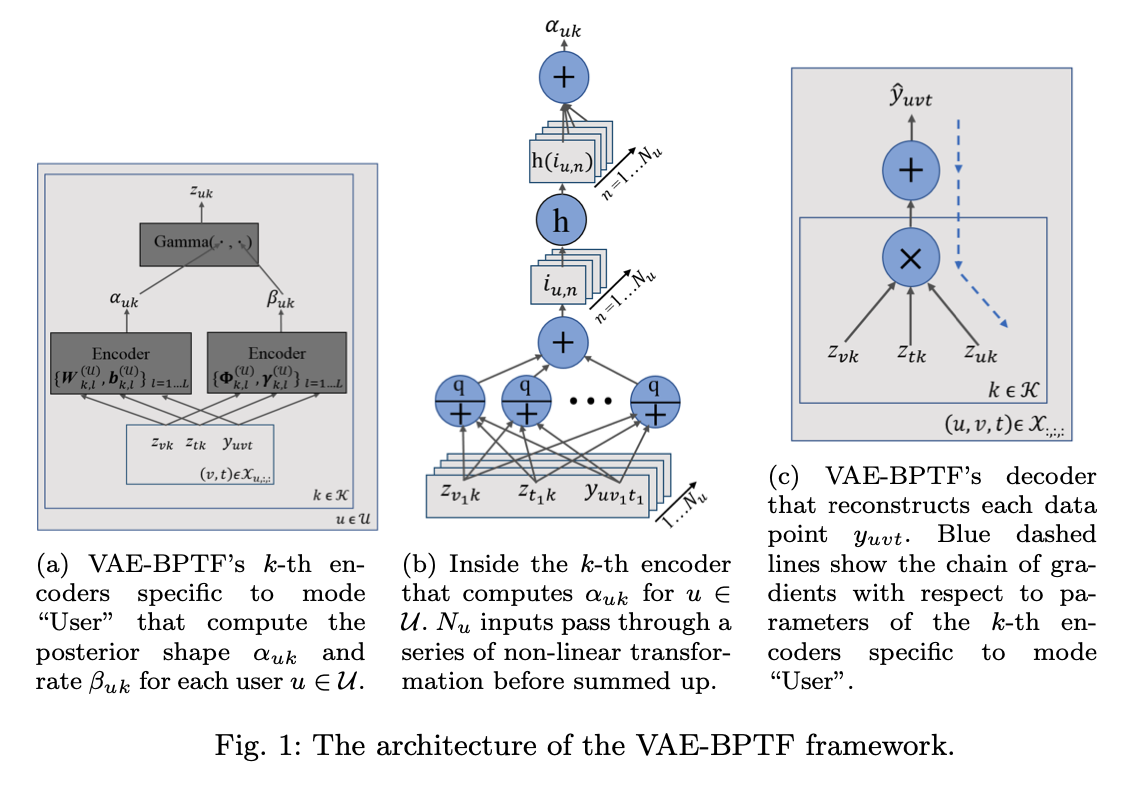kr-kopal150 views
###### Variational Auto-encoder Based Bayesian Poisson Tensor Factorization for Sparse and Imbalanced Count DataSantosh Pandey176 views
###### Video Capsule Endoscopy and Ingestible Electronics: Emerging Trends in Sensors, Circuits, Materials, Telemetry, Optics, and Rapid Reading SoftwareRohit K Ramakrishnan142 views
###### Integrated Photonic Platforms for Quantum Technology: A ReviewOrazio Scarlatella471 views
###### Dynamical Mean-Field Theory for Markovian Lattice Modelspaulclinton137 views
###### [GPT-4] Technical Report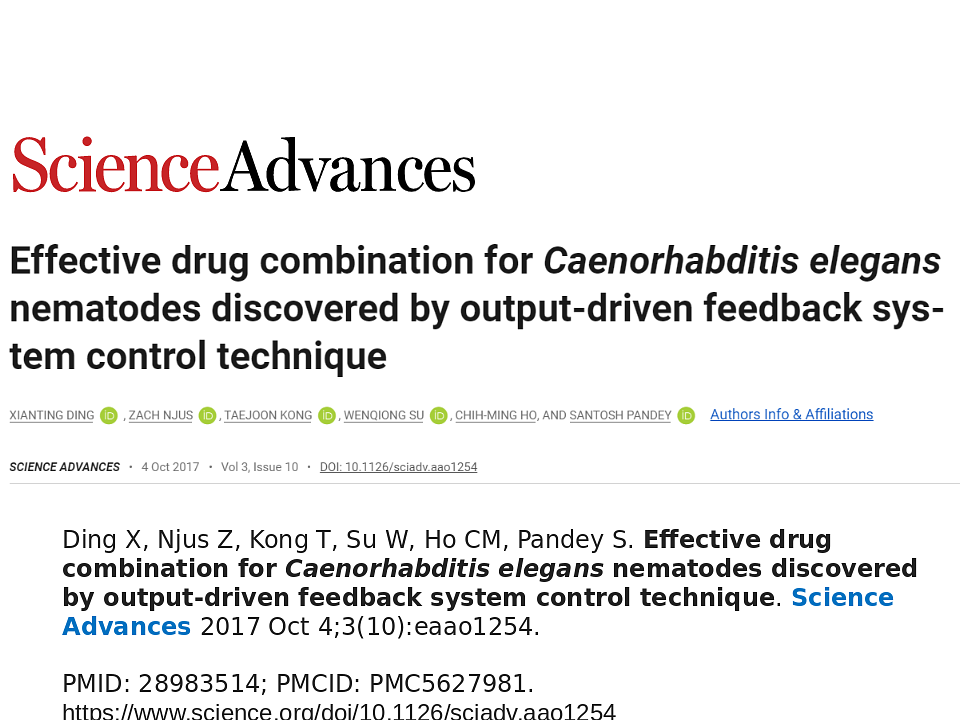Santosh Pandey170 views
###### Multiparameter behavioral analyses provide insights to mechanisms of cyanide resistance in caenorhabditis elegansSantosh Pandey178 views
###### Unidirectional, electrotactic-response valve for Caenorhabditis elegans in microfluidic devices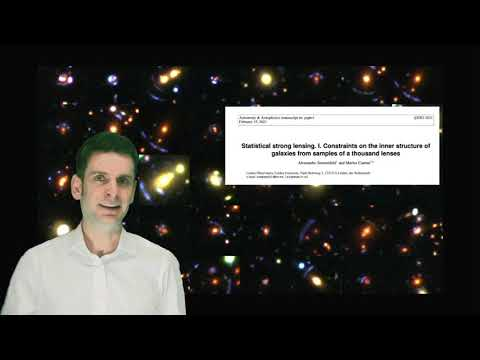Alessandro Sonnenfeld223 views
###### Statistical strong lensing. I. Constraints on the inner structure of galaxies from samples of a thousand lensestaiducnguyen-drexel156 views
###### VideoFACT: Detecting Video Forgeries Using Attention, Scene Context, and Forensic Traces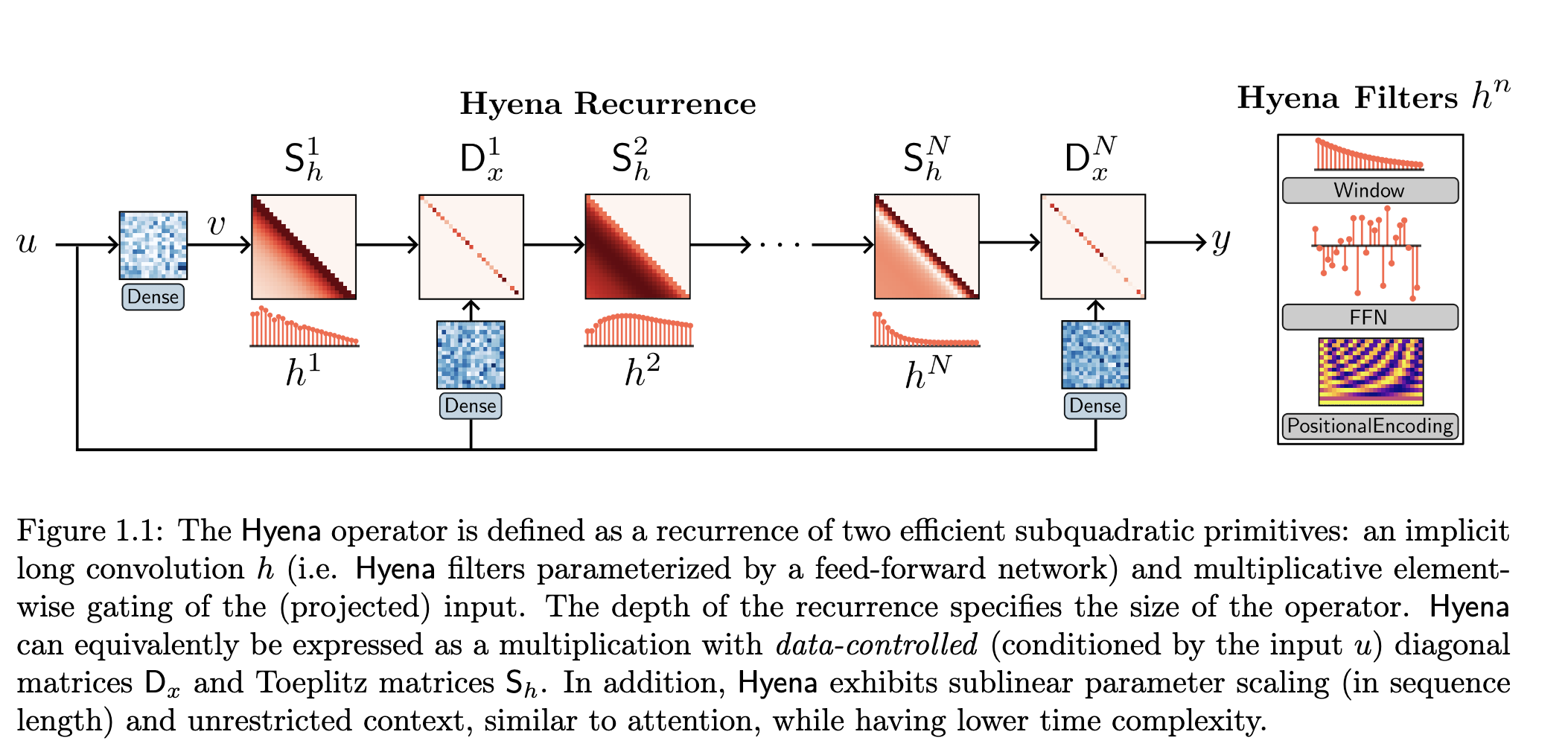paulclinton129 views
###### Hyena Hierarchy: Towards Larger Convolutional Language ModelsArnaud Cedric KAMKOUM41 views
###### The Federal Reserve's Response to the Global Financial Crisis and Its Long-Term Impact: An Interrupted Time-Series Natural Experimental Analysis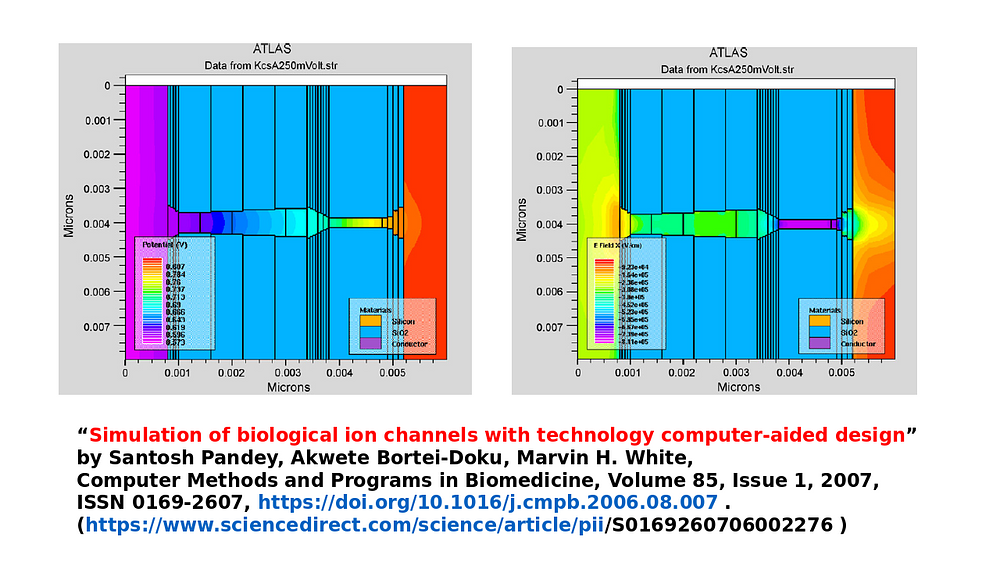Santosh Pandey181 views
###### Simulation of biological ion channels with technology computer-aided designScienceCast Board 84 views
###### A New Gravitational Action For The Trace Anomaly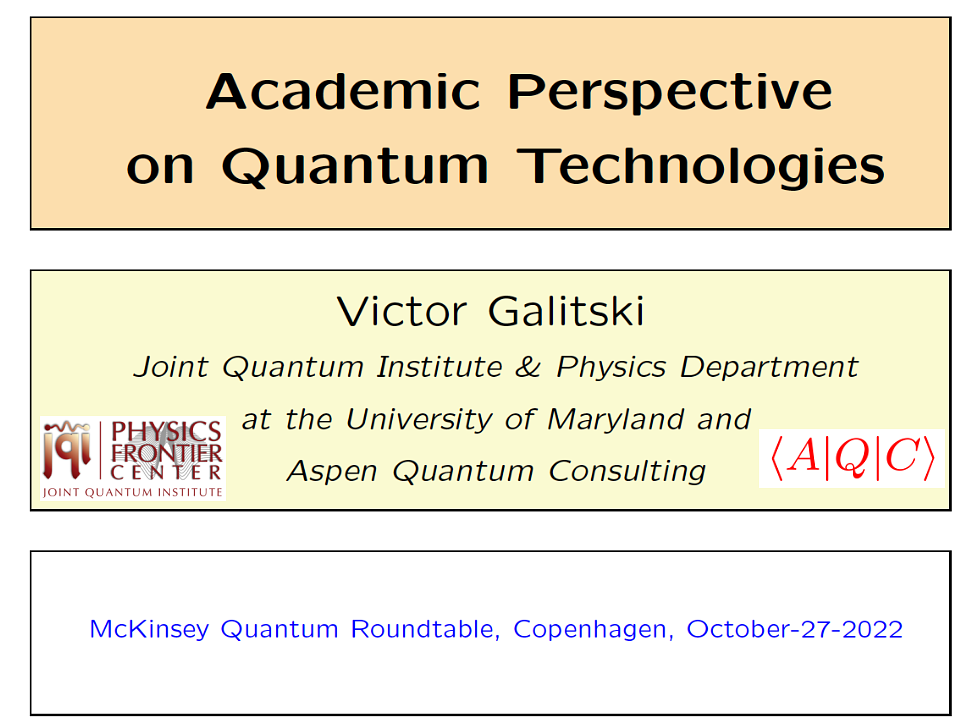AQC team378 views
###### Academic Perspective on Quantum Technologiesnhat-minh-nguyen-111248 views
###### Evidence for suppression of growth of structure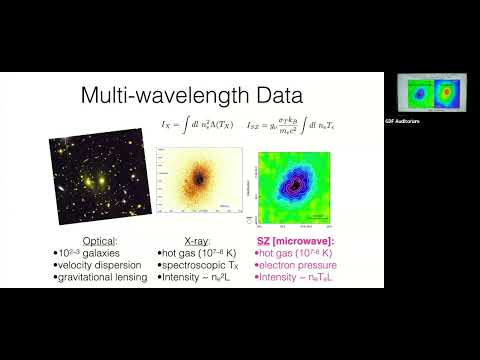Eiichiro Komatsu242 views
###### The Temperature of Hot Gas in the Universe